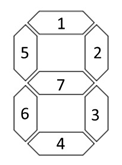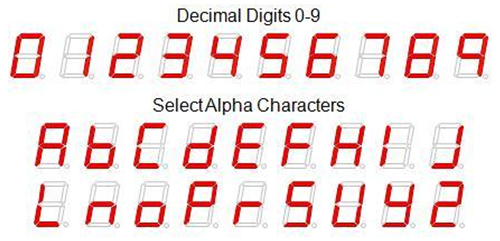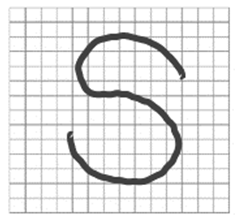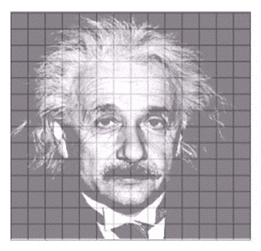Categories

# For the units 6-7 assignment you will be required to build and train

For the units 6-7 assignment you will be required to build and train a neural network to recognize letters of the alphabet and numbers based upon their design using a seven segment display where each segment of the display is one input into the neural network.Using the numbering for the segments in the previous figure as the inputs into your neural network.  You are welcome to use any network design that will accurately solve the problem.   In this case your network must be able to identify the letter or number based upon the pattern of segments.   For example if the segments 1,2,3,4, and 7 are lighted then the pattern would represent the number 3.  The number three must be represented as a binary number that is the output of the neural network.

Not all of the letters of the alphabet can be accurately recognized.  The following chart represents the numbers and letters that your network must be able to recognize with the exception of letters S and Z which cannot be distinguished from the numbers 2 and 5.The output of your network will be the ASCII code of the letter or number defined in the following list and represented as binary.  Since the largest number is 72 you will require 7 outputs to represent any of the 7 segment numerals.

Character       ASCII       Neural Network Output (Binary)

0                  48                0110000

1                  49                0110001

2                  50                0110010

3                  51                0110011

4                  52                0110100

5                  53                0110101

6                  54                0110110

7                  55                0110111

8                  56                0111000

9                  57                0111001

A                 65                1000001

B                 66                1000010

C                 67                1000011

D                 68                1000100

E                 69                1000101

F                 70                1000110

H                 72                1001000

We are using a 7 segment display to recognize letters and numbers, however it is important to know that we could use the same approach to recognize virtually anything.   We are using a 7 segment display because our simulated neural network only has the capacity to accommodate 10 input values.  However if we had more input values we could recognize any handwritten letter or number.  Consider the following examples example.  Assume that we had a matrix that was 16 x 16 (total of 256 point) and each of the points were an input into our neural network.  Further assume that we could overlay a hand written letter or number onto this matrix.  Every pixel that was colored by the letter would have a value of 1 and any pixel that was not colored would have a value of 0.Using this as input we could easily train a neural network to ‘recognize’ various hand written letters.  As the amount of training increases the accuracy of the network to detect similar letters that might not have the exact same shape (but a similar one) would increase.

Let’s consider another example.   Assume that we had a neural network and instead of the inputs being 1 or 0, we had the ability to determine a value between 0 and 1 as input where 0 was the color white and 1 the color black and shades of grey as values in between 0 and 1.Using this approach we could train the neural network to recognize faces, people, and objects … such as Albert Einstein here.  In practice, facial recognition software will typically limit the amount of ‘features’ that are used as input to the neural network to areas that have the greatest predictive power such as the position of the eyes, nose, mouth, and perhaps chin, but the principle remains the same as the network that we will build to recognize the letters in the 7 segment display.

To complete this assignment, you will need to download and install the Basic Prop neural network simulator.  Basic Prop is distributed as an executable Java Jar file.  You can either execute the file on your local computer or you can access the simulator in the Virtual Computing Lab.   To run the simulator on your local computer you will need to have the Java JRE (java runtime environment) version 1.5 or greater installed on your computer.

The simulator can be downloaded from the basic prop website at the following URL: http://basicprop.wordpress.com/2011/12/21/introducing-basic-prop-a-simple-neural-networ/

As part of the assignment you will need to create a pattern file for the 7 segment display input data.  You can see an example of a pattern file on the basic prop website.

I have provided an example of a pattern file below.  In this case I have set up the pattern file to teach the neural network to add two binary numbers.  Notice that the first set of digits is the input value in binary.  The second set of digits is the output value of the network.  Notice how in the first set of digits I have 0 0 0 0 1 0 0 0 0 0 1 as input.  In this example which adds two numbers together, I have defined the input as two binary numbers each 5 digits in length (in the first example the two numbers are 1 in binary).  The second set of digits are the output of the network in the example it would be 2 in binary (0 0 0 1 0) ….1 plus 1 is 2.

The network is then ‘taught’ to add the two numbers together.

Number of patterns = 3

Number of inputs = 10

Number of outputs = 5

[Patterns]

0 0 0 0 1 0 0 0 0 1    0 0 0 1 0

0 0 0 1 0 0 0 0 0 1    0 0 0 1 1

0 0 0 1 1 0 0 0 1 0    0 0 1 0 1

Another example is using the neural network to process logic patterns such as AND, OR, and the XOR. You can download the pattern file for AND logic and use the same file format for a network that can solve the 7 segment display problem (you could also cut and paste my add numbers pattern file from above).   When you have created the pattern file (the pattern file defines the number of inputs and the outputs in the problem and the number of test cases you can use it to train and test your neural network.

Using the simulator you will need to design a network, load the pattern file, and then train the network.  You should experiment with your network design to make sure that your network and solve all of the test cases.  If your network is not solving all of the cases, then you should alter the design of the network and train the network again.When you have workable network design that solves all of the cases:

• You should save your network, capture a screen shot of your simulator with the completed solution, and save your pattern file.

• You must report on the results of testing your network after it has been trained.

• You must include the average per pattern error metric as part of your testing results.

• Your error should be under 5% in order for the network to be acceptable.

• You must report the number of training steps that your network required.

• You should report the metrics chosen in basic prop for training your network report the defaults if you do not change them.

• You should report these metrics for EACH network that you test identifying the network that you selected as the solution for your assignment.

• You should also use the feature in basic prop to save your weights to a file.

• All of these items should be submitted as part of your assignment.

• You should also write a short paper explaining the process that you went through to develop your network including any network designs that were unable to accurately determine what each input character was and the reasons that you discovered the network design was not successful.

NOTE: You will have 2 weeks to compete this assignment; it will be due at the end of Week/Unit 7.

### QUICK QUOTE

Approximately 250 words
\$12